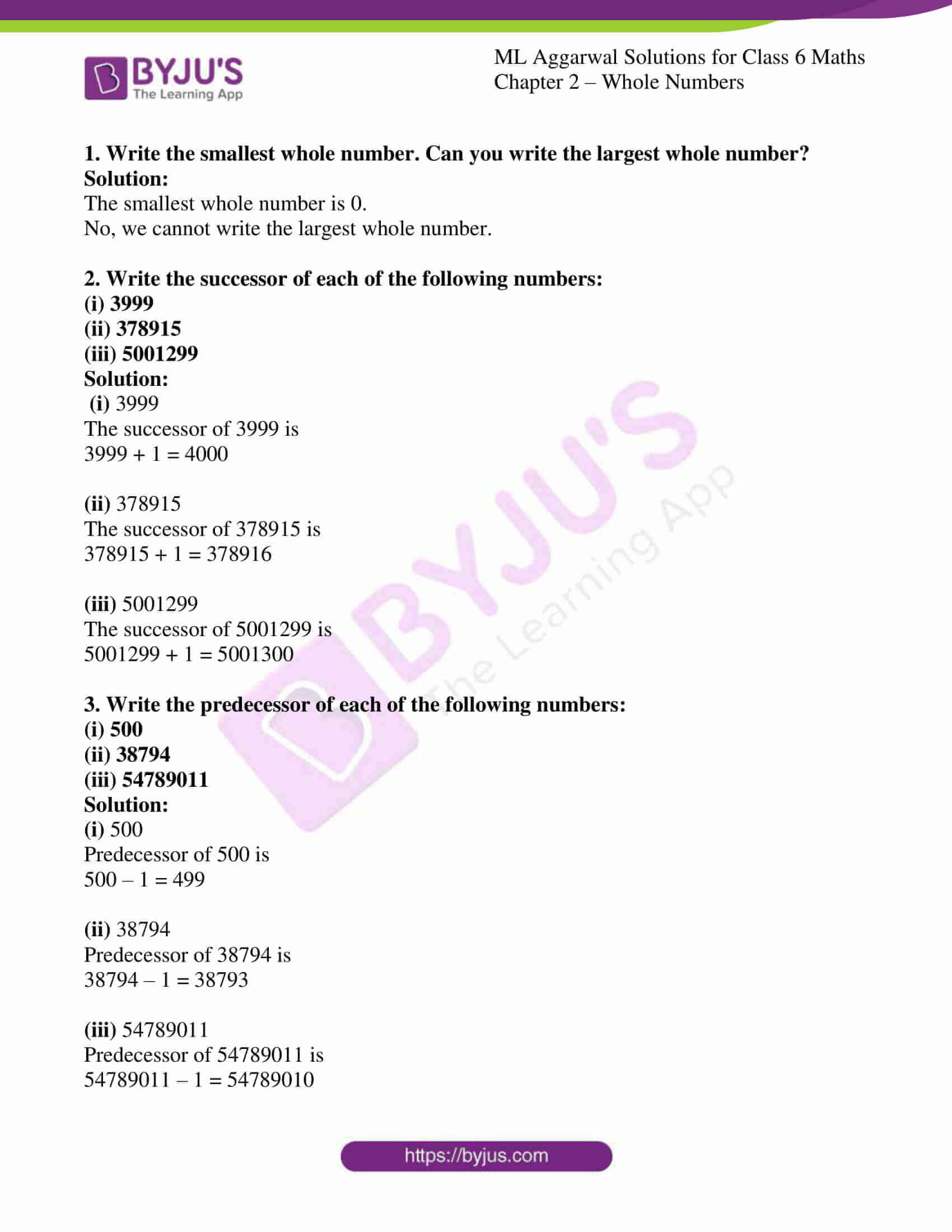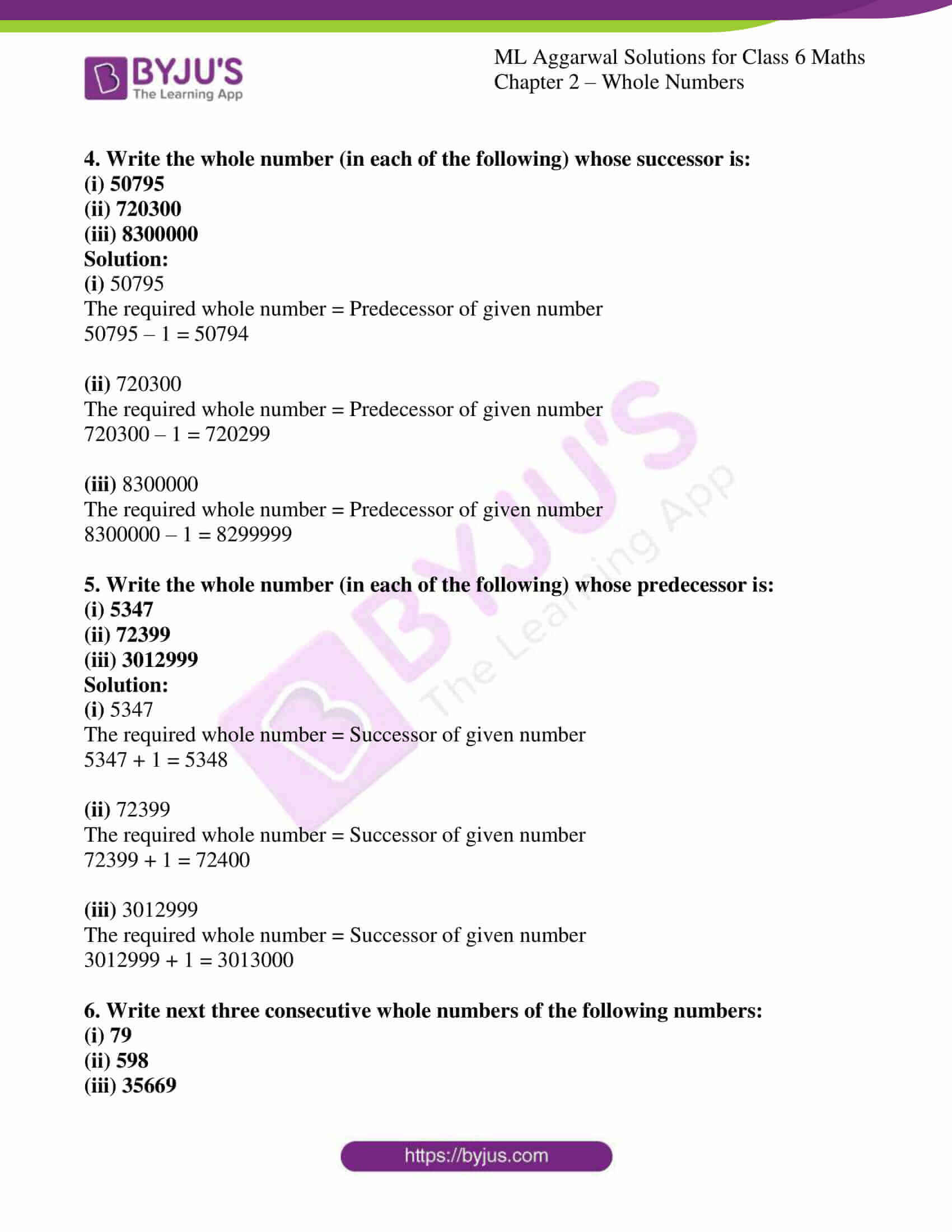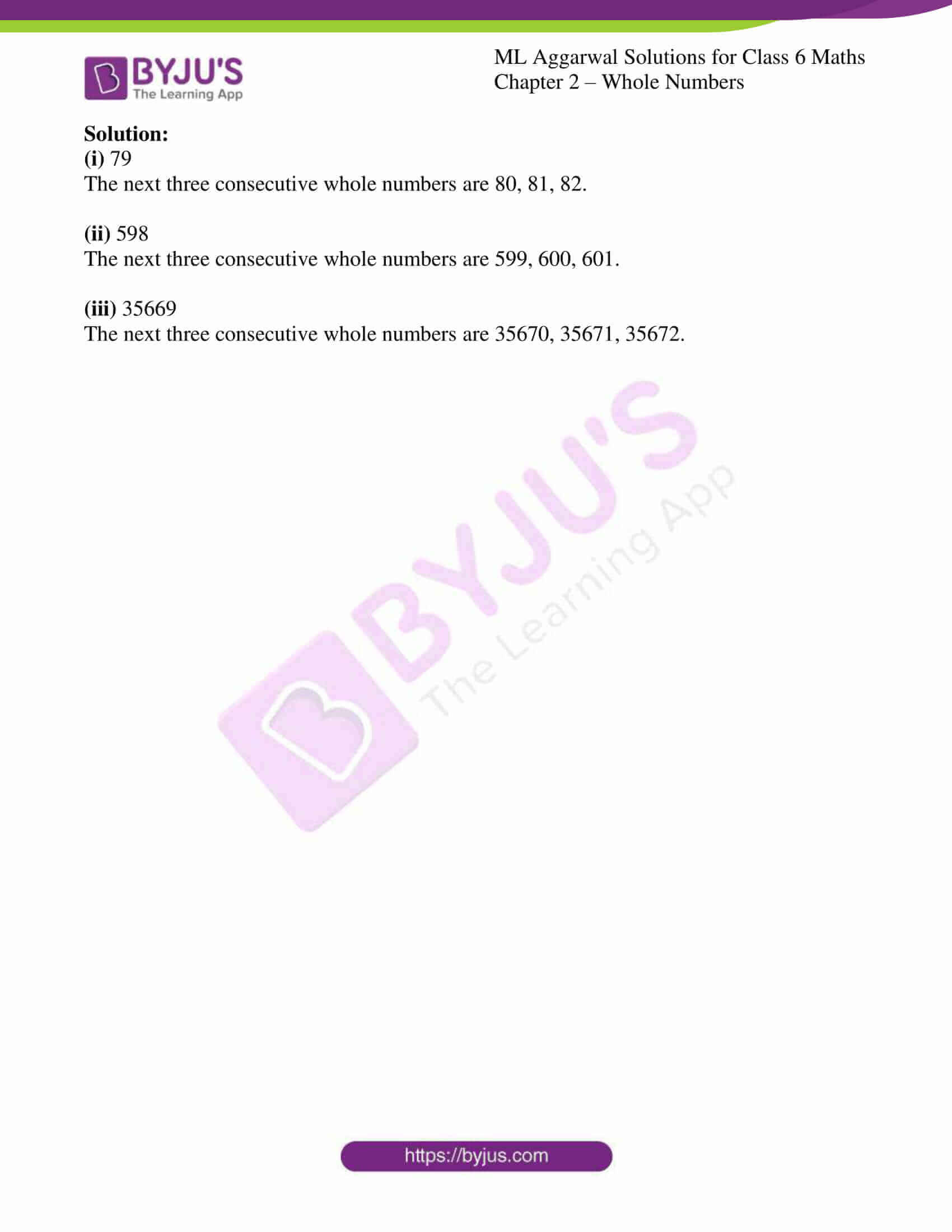# ML Aggarwal Solutions for Class 6 Maths Chapter 2 - Whole Numbers

ML Aggarwal Solutions for Class 6 Maths Chapter 2 – Whole Numbers are provided here to help students prepare for their exams and score well. This Chapter mainly deals with problems based on Whole Numbers. The concepts are explained in detail and solutions are prepared by our expert faculty team in the best possible ways students can understand. Students are advised to refer to ML Aggarwal Solutions as reference material, which helps in boosting their confidence level to achieve high marks in their exams.

Chapter 2 – Whole Numbers provides solutions to questions related to each and every topic present in this Chapter. Students can refer and easily download the PDF of ML Aggarwal Class 6 Solutions for free, from the links given below.

## Download the PDF of ML Aggarwal Solutions for Class 6 Maths Chapter 2 – Whole Numbers### Access answers to ML Aggarwal Solutions for Class 6 Maths Chapter 2 – Whole Numbers

1. Write the smallest whole number. Can you write the largest whole number?
Solution:

The smallest whole number is 0.

No, we cannot write the largest whole number.

2. Write the successor of each of the following numbers:
(i) 3999
(ii) 378915
(iii) 5001299
Solution:

(i) 3999

The successor of 3999 is

3999 + 1 = 4000

(ii) 378915

The successor of 378915 is

378915 + 1 = 378916

(iii) 5001299

The successor of 5001299 is

5001299 + 1 = 5001300

3. Write the predecessor of each of the following numbers:
(i) 500
(ii) 38794
(iii) 54789011
Solution:

(i) 500

Predecessor of 500 is

500 – 1 = 499

(ii) 38794

Predecessor of 38794 is

38794 – 1 = 38793

(iii) 54789011

Predecessor of 54789011 is

54789011 – 1 = 54789010

4. Write the whole number (in each of the following) whose successor is:
(i) 50795
(ii) 720300
(iii) 8300000
Solution:

(i) 50795

The required whole number = Predecessor of given number

50795 – 1 = 50794

(ii) 720300

The required whole number = Predecessor of given number

720300 – 1 = 720299

(iii) 8300000

The required whole number = Predecessor of given number

8300000 – 1 = 8299999

5. Write the whole number (in each of the following) whose predecessor is:
(i) 5347
(ii) 72399
(iii) 3012999
Solution:

(i) 5347

The required whole number = Successor of given number

5347 + 1 = 5348

(ii) 72399

The required whole number = Successor of given number

72399 + 1 = 72400

(iii) 3012999

The required whole number = Successor of given number

3012999 + 1 = 3013000

6. Write next three consecutive whole numbers of the following numbers:
(i) 79
(ii) 598
(iii) 35669
Solution:

(i) 79

The next three consecutive whole numbers are 80, 81, 82.

(ii) 598

The next three consecutive whole numbers are 599, 600, 601.

(iii) 35669

The next three consecutive whole numbers are 35670, 35671, 35672.